U.S. Dept. of Commerce / NOAA / OAR / PMEL / Publications

## On the Variability of Winds, Sea Surface Temperature, and Surface Layer Heat Content in the Western Equatorial Pacific

### 5. Surface Turbulent Fluxes, Vertical Advection, and Entrainment

Figure 7 shows time series of wind speed (|U|), zonal wind pseudostress (|U|U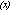), and pseudowork (|U|) from the mooring at 0°, 165°E for December 1986 to October 1987. In each case, estimates were first computed from hourly data, then averaged to daily values. Ignoring the weak wind speed dependence of Cand Cfor the range of speeds shown in Figure 7, turbulent air-sea heat exchange will be proportional to |U|, Ekman pumping proportional to |U|U, and wind work proportional to |U|. The SST and 50-m temperature from the equatorial mooring are also shown in Figure 7.

##### If your browser cannot view the following table correctly, click this link for a GIF image of Table 1.
Wind Zonal Speed Mean 4.5 9.7 184 29.2 (29.2) (29.0) Standard deviation 2.0 24.8 227 0.31 (0.22) (0.11) Numbers in parentheses for SST and T(50 m) are statistics for December 13, 1986 to May 31, 1987, when the surface layer extended to at least 50-m depth.

Table 1 summarizes the means and standard deviations of the time series in Figure 7. The mean wind speed is only 4.5 m s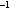and the mean zonal pseudostress is eastward at 9.7 ms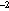(for a constant Cof 1.2 × 10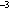, this pseudostress translates into a mean zonal stress of about 0.014 N m). Standard deviations in both of these variables are also small: 2.0 m sfor |U| and 24.8 msfor |U|U(equivalent to 0.036 N m). SST is high on average (29.2°C), with a standard deviation of 0.31°C. Temperature at 50 m is 29.0°C on average, with a standard deviation two times smaller than the SST standard deviation during the first part of the record, when 50 m is located in the surface layer.

Fig. 8. Cross correlations between SST and wind speed, zonal wind pseudostress, and wind pseudowork. Cross correlation extrema (r) and the lag (in days) at which they occur are shown. In each case, the lag indicates that cold SST follows high winds by 1 day. The 95% confidence limits for the null hypothesis of uncorrelated variability are indicated by horizontal bars on the abscissa.

Figure 8 indicates the cross correlations of the three wind constructs with SST are significantly nonzero at the 95% level of confidence and range between -0.43 (for zonal pseudostress) and -0.52 (for wind speed). The negative correlation indicates that high wind speed, high wind work, and westerly wind stress (relative to the mean) are associated with cool SST; low wind speed, low wind work, and easterly wind stress (relative to the mean) are associated with warm SST. In each case the maximum cross correlation occurs with SST lagging the winds by 1 day, consistent with the atmosphere forcing the ocean on these time scales. Although not presented, the magnitude of the cross correlation between meridional wind pseudostress and SST is generally0.2 over the range of lags shown in Figure 8.

Fig. 9. Coherence and phase spectra for SST and wind speed, zonal wind pseudostress, and wind pseudowork at 0°, 165°E for the period December 13, 1986, to October 14, 1987. Positive phase indicates that high winds lead high SST, and negative phase indicates that high winds lead low SST. Horizontal lines superimposed on coherence estimates indicate 95% confidence limits for the null hypothesis of incoherent variability, based on five (15) frequency band averages at periods longer (shorter) than about 8 days.

Another way to view these relationships is in the frequency domain. Figure 9 shows the coherence and phase as a function of frequency between the three wind constructs and SST. Two bands of high and statistically significant coherence are evident. These are at periods between approximately 3-8 days and 30-60 days; to a lesser extent, variations at 10-20 days are also coherent. Consistent with the cross-correlation statistics, the phase in most frequency bands is between -90° and -180°, implying that maxima in the winds are followed by cold SST and minima are followed by warm SST. Coherence at periods shorter than 20 days may be related to westerly wind burst activity. Coherence at periods of 30-60 days could be related to atmospheric fluctuations associated with Madden and Julian  waves.

Note that although there are differences between the coherence and phase diagrams in Figure 9 and the cross-correlation analysis summarized in Figure 8, the statistical relationships of the three wind constructs with SST share many similarities; indeed, wind speed, zonal wind pseudostress, and wind work are highly correlated among themselves (0.61-0.90). Hence without further analysis one cannot unambiguously identify which of the processes related to the wind field are most important in affecting SST.

Fig. 10. Coherence amplitude as a function of depth averaged over periods of approximately 3-8 days (encompassing 36-65 frequency bands depending on depth) for SST and wind speed, zonal wind pseudostress, and wind pseudowork at 0°, 165°E. The 95% confidence limits for the null hypothesis of incoherent variability are indicated by the dashed lines. Note that the temperature record lengths for this analysis were chosen such that the statistics were stationary; for example, the period June-October 1987 was excluded at 50 m and 75 m. Shown on the right are the mean temperature profile (solid curve) and the standard deviation of temperature variations at periods of approximately 3-8 days (dashed curve) for December 13, 1986, to October 14, 1987.

Fig. 11. Coherence amplitude as a function of depth averaged over periods of approximately 30-60 days (that is 4-6 frequency bands, depending on depth) for SST and wind speed, zonal wind pseudostress, and wind pseudowork at 0°, 165°E. The 95% confidence limits for the null hypothesis of incoherent variability are indicated by the dashed lines. Shown on the right are the mean temperature profile (solid curve) and the standard deviation of temperature variations at periods of approximately 30-60 days (dashed curve) for December 13, 1986, to October 14, 1987.

Figure 10 shows coherence amplitude as a function of depth in the 3- to 8-day period band. Also shown in Figure 10 is the mean temperature profile for December 1986 to October 1987. Wind speed, wind stress, and wind work are all significantly coherent with temperature variations between 1 and 10 m. Coherence drops rapidly below these depths, however, and is statistically insignificant at the 95% level in the lower part of the surface layer between 50 and 75 m. In the thermocline, wind speed and wind work are generally incoherent with temperature fluctuations; on the other hand, zonal pseudostress shows a clear pattern of coherence between 125 and 300 m. The coherence profiles for the 10- to 20-day and 30- to 60-day period bands are generally similar to those shown in Figure 10. Figure 11, for example, shows the coherence as a function of depth for wind speed, zonal pseudostress, and wind work in the 30- to 60-day band. In each case the coherence is high at the surface, then falls off rapidly to relatively low and/or insignificant values. Only in the case of zonal pseudostress does significantly nonzero coherence appear consistently in the thermocline.

Fig. 12. Coherence amplitude and phase as a function of depth averaged over periods of approximately 3-8 days (encompassing 36-65 frequency bands, depending on depth) for SST and zonal wind pseudostress at 0°, 165°E. The 95% confidence limits for the null hypothesis of incoherent variability are indicated by the dashed curve.

Figure 12 shows that the phase of zonal pseudostress coherence in the 3- to 8-day band is such that warm thermocline temperatures lag relative westerly winds by about 90° and cold thermocline temperatures lag relative easterly winds by the same amount. This is consistent with the Ekman pumping hypothesis for thermocline variations discussed in section 4. However, phase changes by 180° across the base of the surface layer, indicating that temperature variations are of opposite sign in the surface layer and thermocline. A similar phase change occurs at lower frequencies as well. Vertical advection from the thermocline is therefore not likely to be the principal mechanism controlling SST on these time scales, as was earlier suggested in the discussion of Figure 5a. Likewise, the rapid decrease in coherence with depth below 10 m in Figures 10 and 11 suggests that entrainment from the thermocline is not, in general, important in controlling SST. One would expect coherence between temperature and |U|to be much higher at 50-75 m if the thermocline were the source of cold water for the surface layer. This leaves turbulent surface fluxes proportional to |U| as the most likely candidate for affecting SST and surface layer heat content.

Fig. 13. Time series ofH/t for depth ranges of (a) 0-10 m for the period December 13, 1986, to July 20, 1987, and (b) 0-75 m for the period December 13, 1986, to May 31, 1987. The standard deviation of heat content variations (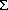) and cross correlation with wind speed at zero lag (r) are shown.

Further evidence to support the role of wind-related surface turbulent heat flux variations can be found in an examination of upper ocean heat content (defined as H = pChT) from the 0°, 165°E mooring. Figure 13 shows time series ofH/t estimated using centered differences over two depth ranges: 0-10 m (where coherence with the wind speed is highest) and 0-75 m (the average depth of the surface layer). The calculation for 0-75 m is shown only through the end of May 1987, because of the nonstationarity of the time series during the second half of the record when the upper portion of the thermocline reaches depths of 50-75 m (see Figures 3 and 7). The standard deviation ofH/t() and the cross correlation at zero lag (r) ofH/t and |U| also appear in Figure 13. For both depth ranges the cross correlations are significantly nonzero (with 95% confidence) and are higher than for either pseudostress or for wind work.

Variations in heat content within the 0- to 75-m layer are 102 W m. Multiplying this number by 0.32, that is, the magnitude of the correlation betweenH/t and |U|, indicates that heat content changes of approximately 33 W min the upper 75-m surface layer are due to wind speed variations. This estimate is comparable to what would be expected for latent heat flux variations based on (2a) and typical observed parameter values. Specifically, Q35 W mfor wind speed variations of 2.0 m s(Table 1), C, of 1.2 × 10[Large and Pond, 1982] and a typicalq of 5 g kg[Reed, 1985; Liu, 1988].

For comparison, the magnitude of variations in entrainment mixing due to wind work can be estimated from (1) and (5), using a scale analysis similar to that for latent heat flux. For |U|of 227 ms(Table 1), m = 0.4 [Davis et al., 1981; McPhaden, 1982], (T/p)= 3°C mkgat the base of the surface layer (determined from conductivity-temperature-depth (CTD) casts near 0°, 165°E), and h = 75 m, we find thatCwT=5 W m. Other choices of m, h, and (T/p)would result in different estimates of entrainment from the thermocline; however, it is unlikely that a reasonable combination of these parameters would increase our 5 W mestimate by an order of magnitude. Thus we conclude that latent heat flux variations are significantly more important than wind work-related entrainment fluxes from the thermocline in controlling SST and surface layer heat content during the time period of this study.

A comparison of heat content variations over the 0- to 10-m layer and the 0- to 75-m layer indicates that surface cooling associated with Qconverges nonlinearly in the upper 75 m. The product of |r| andover the two depth ranges in Figure 13 implies a standard deviation of 14 W mand 33 W mfor wind-related heat content variations in the upper 10 m and 75 m, respectively. The 19 W mdifference between these two flux estimates represents variability in the depth range 10-75 m. Thus 14 W m(42% of the total) converges in the upper 10 m (13% of the 75-m layer depth) whereas 19 W m(58% of the total) converges between 10 and 75 m (87% of the surface layer depth). Using an integral time scale (t) for wind-speed-related temperature variations of 3-4 days (based on the cross-correlation analysis in Figure 8) and aH/t of 14 W m(0-10 m) and 19 W m(10-75 m), we find the expected magnitude of temperature variations (T ~ tr/Ch) associated with latent heat flux variations in each of these depth ranges to be about 0.1°C and 0.02°C, respectively. The latter temperature is close to the instrumental accuracy of our temperature sensors [e.g., McPhaden et al., 1990] and probably accounts for the insignificant temperature and wind coherences on a pointwise basis for depths greater than 10 m in the surface layer.

Go to previous or next section